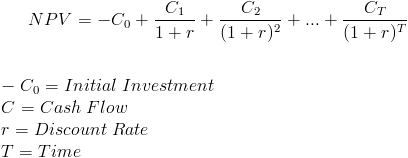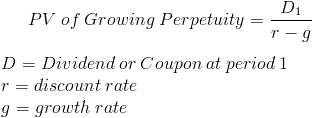# Net present value calculation for both perpetuity and for fix period

NPV
Recent activity on Nov 08, 2018
7.1 k Views

Hi,

What are the formulas for npv for perpetuity and fixed period? I am seeing all sorts of formulas online and cannot tell which is what I need

• Date ascending
• Date descending

Hey there,

If you don't have an MBA, the likelihood of having to use the formula in an interview (at least for MBB) is close to zero. If you did, you'd probably just to have to discount a couple cash flows in the future (i.e. a manual DCF) rather than just calculating the NPV using the NPV formula. If you are applying to different firms, you should check, but again I would be surprised.

Anyways, to answer your question - for a fix period you would just discount each cash flow year by year, see below:In perpetuity, you just divide the cash flow by the (discount rate - the growth in the cash flow):Note that if there is no growth in cash flow, then g=0, and the formula is D/r.